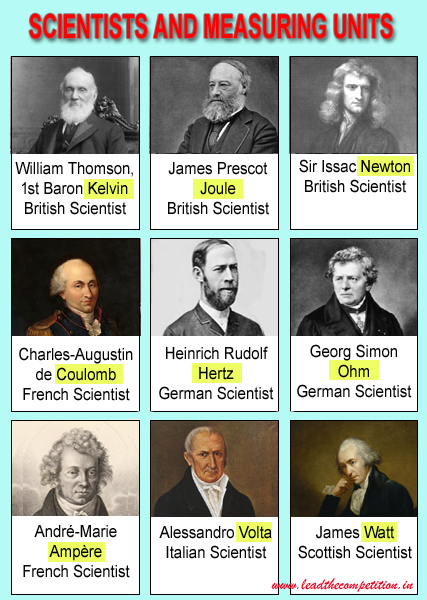# SI Units of Measurement

## Base Units

Physical Quantity Unit of Measurement
LengthMetre
MassKilogram
TimeSecond
Electric CurrentAmpere
Thermodynamic TemperatureKelvin
Amount of SubstanceMole
Luminous IntensityCandela

## Derived Units

Physical Quantity Unit of Measurement
FrequencyHertz
ForceNewton
WeightNewton
PressurePascal
EnergyJoule
WorkJoule
HeatJoule
PowerWatt
Electric ChargeCoulomb
Potential DifferenceVolt
Electromotive ForceVolt
Electric ResistanceOhm
Electric ConductanceSiemens
InductanceHenry
Magnetic FluxWeber
Magnetic Flux DensityTesla

### Scientists and Units of Measurements### Definitions of Some Derived Units

Radian: One radian is the angle subtended at the centre of a circle by an arc that is equal in length to the radius. A semicircle consists of π radians and a circle consists of 2 π radians

Hertz: One hertz being the unit of frequency is one cycle per second.

Newton: One Newton is the force required to produce an acceleration of one metre per second when applied to a mass of one kilogram.

Pascal: One Pascal is equal to 1 Newton per square meter.

Joule: One Joule as the unit of energy or work is equal to the work done by a force of one newton when its point of application moves one metre in the direction of action of the force.

One Joule as the unit of heat energy is defined as the amount of energy required to raise the temperature of one gram of water by one degree Celsius.